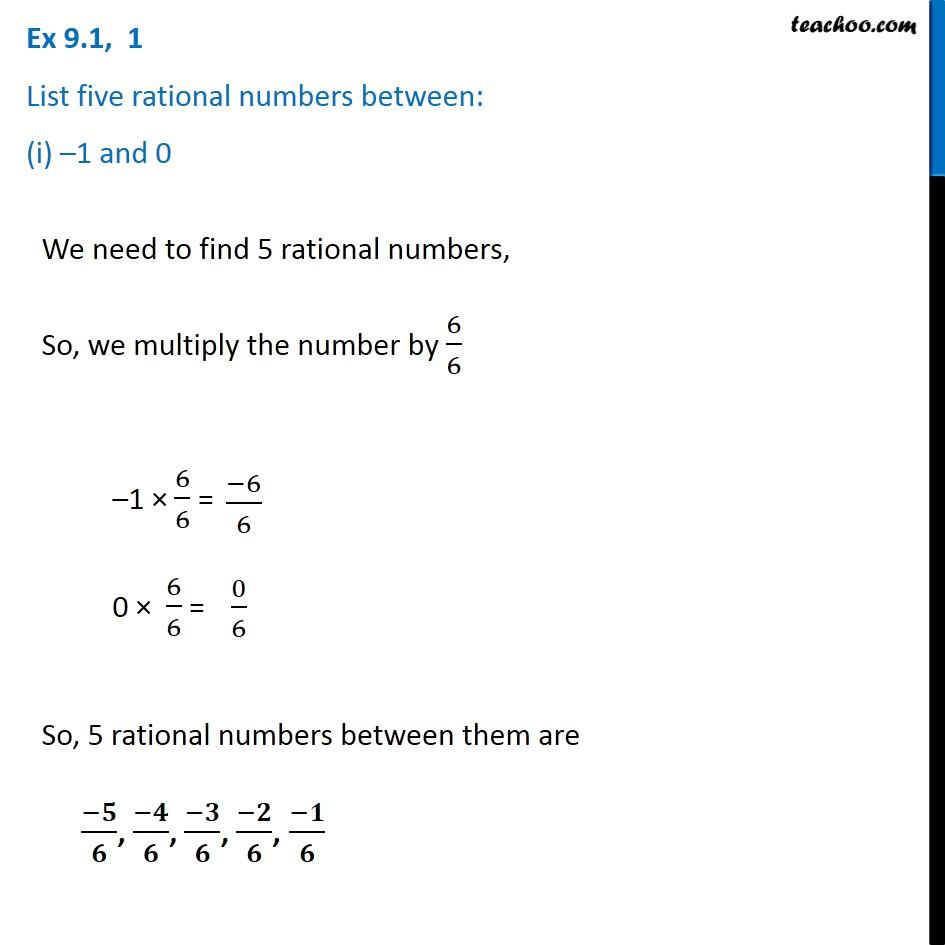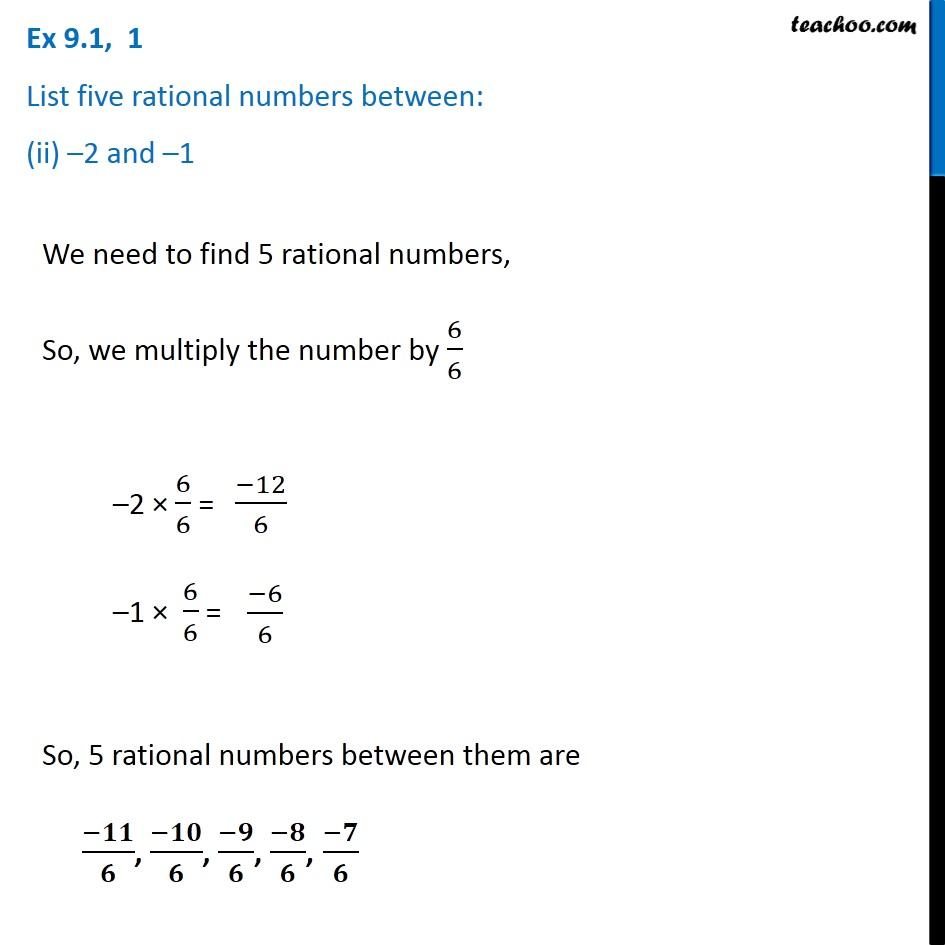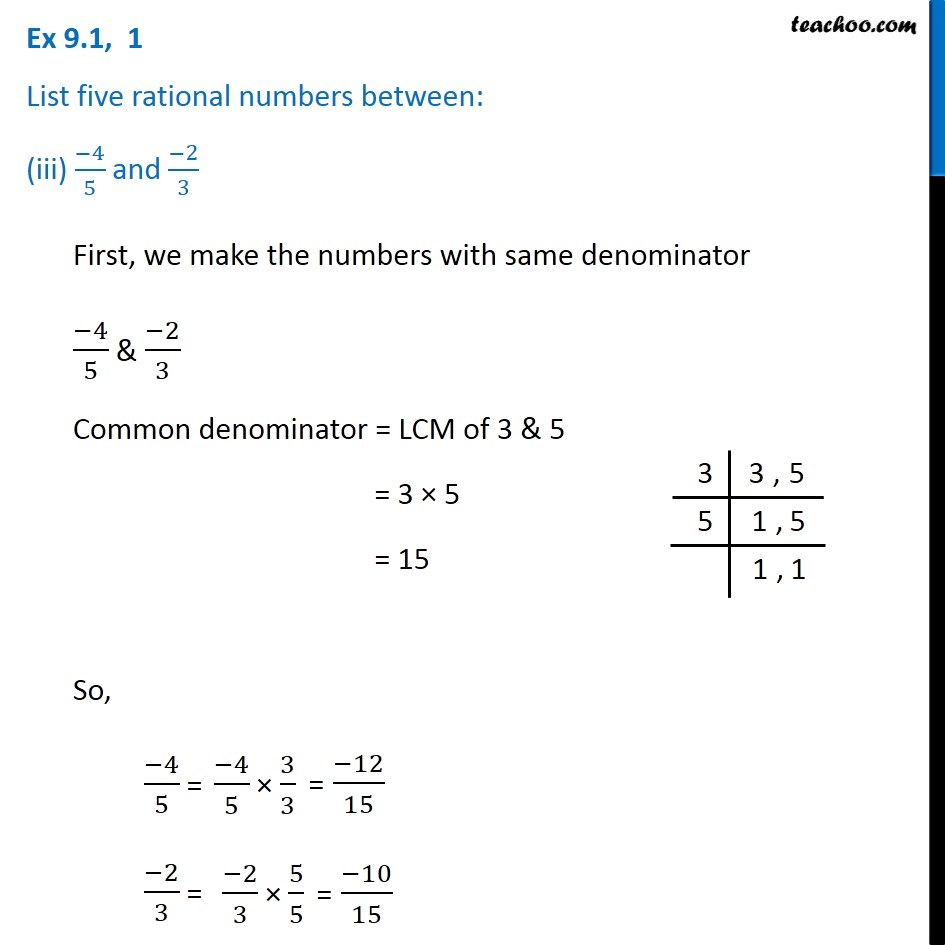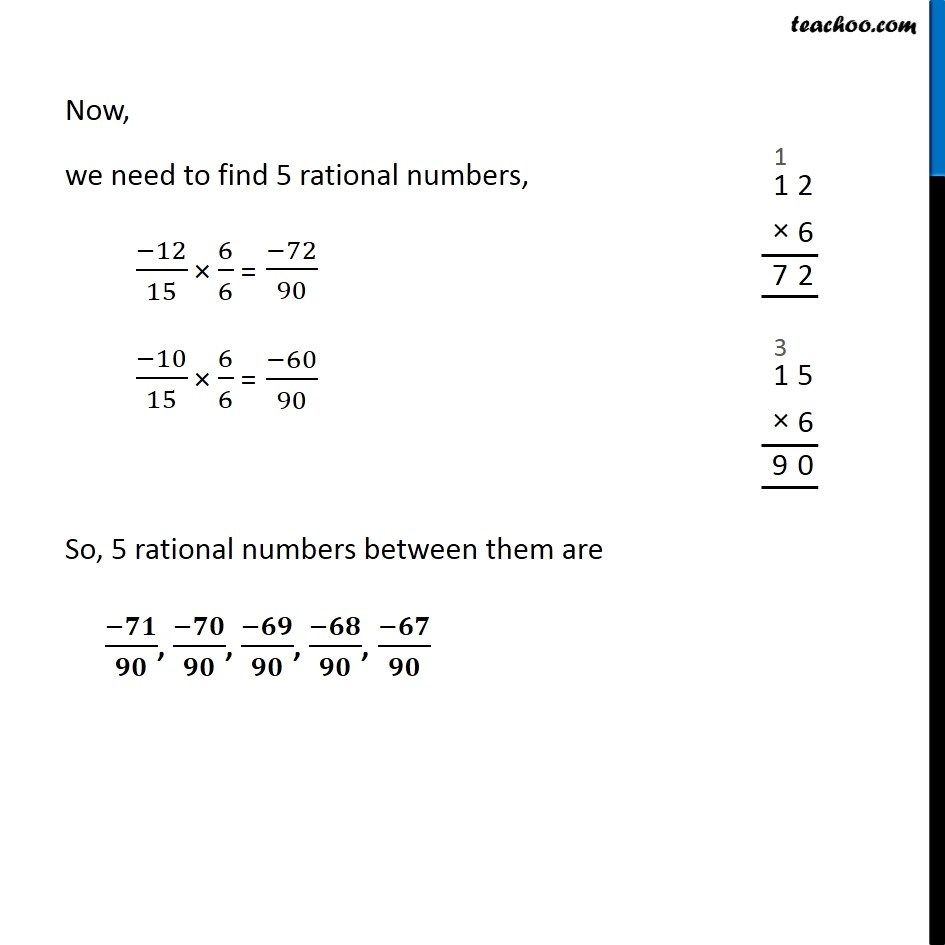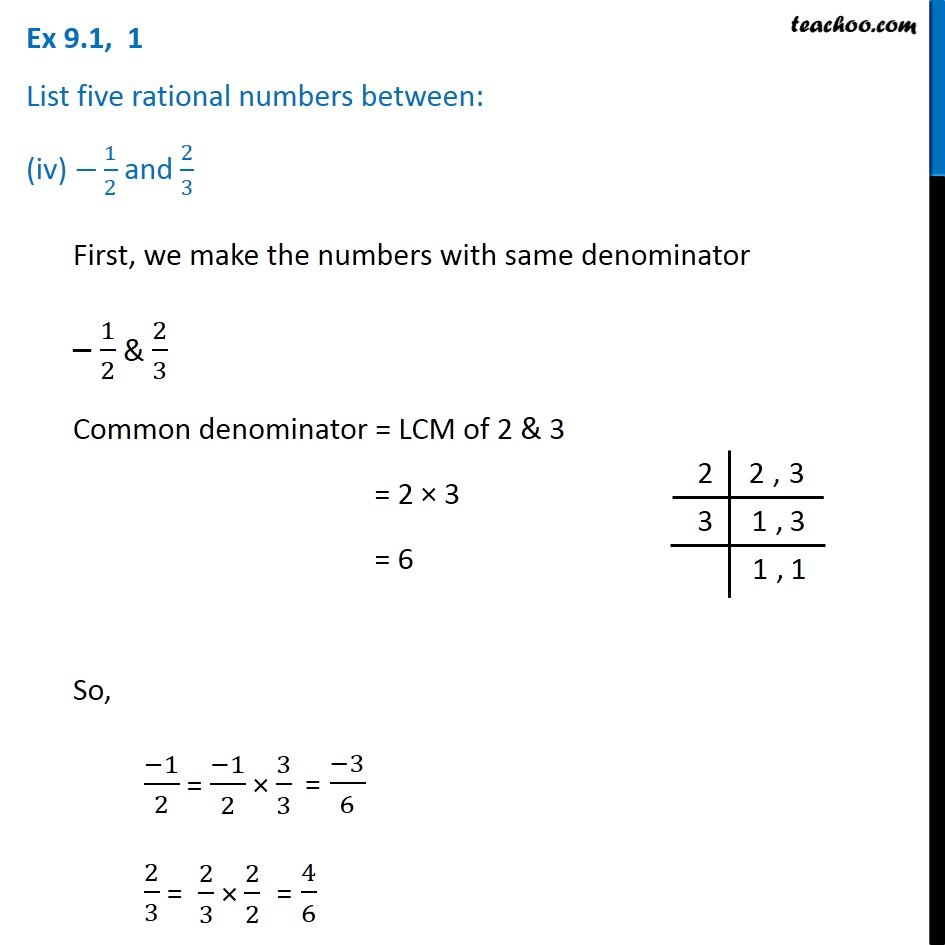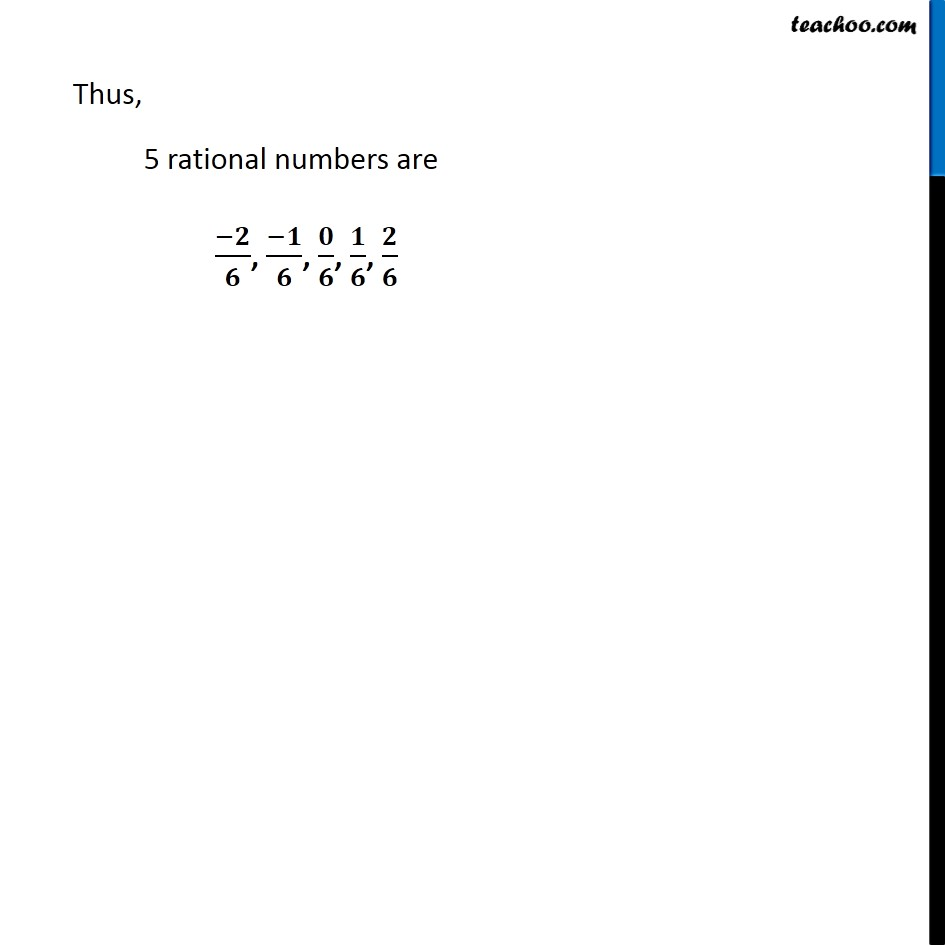1. Chapter 9 Class 7 Rational Numbers
2. Serial order wise
3. Ex 9.1

Transcript

Ex 9.1, 1 List five rational numbers between: (i) –1 and 0 We need to find 5 rational numbers, So, we multiply the number by 6/6 –1 × 6/6 = 6/6 0 × 6/6 = 0/6 So, 5 rational numbers between them are (−𝟓)/𝟔, (−𝟒)/𝟔, (−𝟑)/𝟔, (−𝟐)/𝟔, (−𝟏)/𝟔 Ex 9.1, 1 List five rational numbers between: (ii) –2 and –1 We need to find 5 rational numbers, So, we multiply the number by 6/6 –2 × 6/6 = –1 × 6/6 = So, 5 rational numbers between them are (−𝟏𝟏)/𝟔, (−𝟏𝟎)/𝟔, (−𝟗)/𝟔, (−𝟖)/𝟔, (−𝟕)/𝟔 Ex 9.1, 1 List five rational numbers between: (iii) (−4)/5 and (−2)/3 First, we make the numbers with same denominator (−4)/5 & (−2)/3 Common denominator = LCM of 3 & 5 = 3 × 5 = 15 So, (−4)/5 =(−4)/5 × 3/3 = (−12)/15 (−2)/3 = (−2)/3 × 5/5 = (−10)/15 Now, we need to find 5 rational numbers, (−12)/15 × 6/6 =(−72)/90 (−10)/15 × 6/6 =(−60)/90 So, 5 rational numbers between them are (−𝟕𝟏)/𝟗𝟎, (−𝟕𝟎)/𝟗𝟎, (−𝟔𝟗)/𝟗𝟎, (−𝟔𝟖)/𝟗𝟎, (−𝟔𝟕)/𝟗𝟎 Ex 9.1, 1 List five rational numbers between: (iv) −1/2 and 2/3 First, we make the numbers with same denominator – 1/2 & 2/3 Common denominator = LCM of 2 & 3 = 2 × 3 = 6 So, (−1)/2 =(−1)/2 × 3/3 = (−3)/6 2/3 = 2/3 × 2/2 = 4/6 Thus, 5 rational numbers are (−𝟐)/𝟔, (−𝟏)/𝟔, 𝟎/𝟔, 𝟏/𝟔, 𝟐/𝟔

Ex 9.1